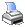﻿ MCL Reference > Information about a Start > HrSectMargLensX( ), JrSectMargLensX( ), SrSectMargLensX( )
 Navigation:  MCL Reference > Information about a Start > HrSectMargLensX( ), JrSectMargLensX( ), SrSectMargLensX( )If the 1st argument is omitted, the functions return the margins behind the fastest horse of all sections in a character string composed of a list of decimal numbers (identical to HrSectMargLens( ), JrSectMargLens( ), SrSectMargLens( )). If a number if passed into the 1st argument, the margins behind the fastest horse of the section will be returned in numeric values. However, for the fastest horse, the length which leads the second horse will be returned in a negative number.

Syntax

HrSectMargLensx(nSection,nBackRaces,'fCondition',cDefaultVal,cIncluding)

JrSectMargLensx(nSection,nBackRaces,'fCondition',cDefaultVal,cIncluding)

SrSectMargLensx(nSection,nBackRaces,'fCondition',cDefaultVal,cIncluding)

Arguments

 • If nSection is omitted or equals to 0, margin behind the leading horse of all sections will be returned in a character string composed of a list of decimal numbers.
 • When nSection is a positive number, it is a section number counting from the starting point (i.e., 1 for the first section and 2 for the second, etc.). The margin behind the leading horse for the section will be returned as a numeric value.
 • When nSection is a negative number, it is a section number counting from the finishing point (i.e., 1 for the last section and 2 for the 2nd last, etc.). The margin behind the leading horse for the section will be returned as a numeric value.

See Arguments of MCL functions for details about other arguments.

Returns

Numeric (decimal=2)